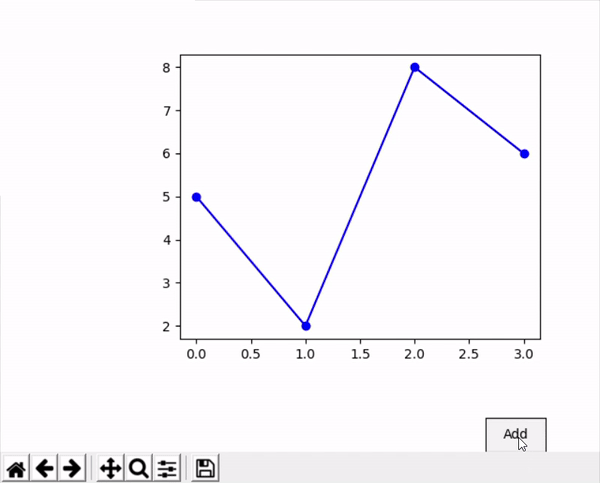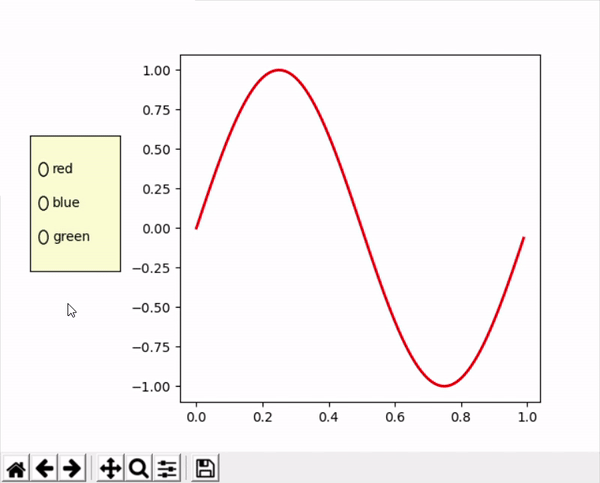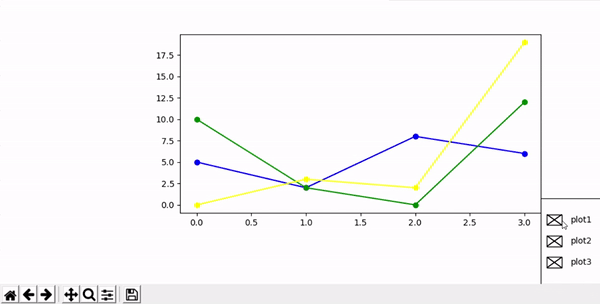# Matplotlib – Button Widget

• Difficulty Level : Easy
• Last Updated : 30 Aug, 2021

In Matplotlib a button is one of the important widgets by which we can perform various operations. They are mostly used for making a good functional graph having different properties. There are three types of buttons

• Button
• Check Buttons

In this article, we will learn how to use different buttons in the matplotlib plot. For this, we will use some data, plot a graph, then form a button and use it. Let’s understand buttons one by one with the help of some examples.

### Simple Button

This is a simple button that is responsible for performing only one function.

Syntax: simple_button=Button()

Parameters:

• ax– defines the axes where the button should be located
• label– the name that we want on the button
• color– color of the button
• hover color– color of the button when it gets clicked

In this example, we will create a simple button, and we will use this button to add one more line to the existing graph.

## Python3

 `# importing libraries``import` `numpy as np``import` `matplotlib.pyplot as plt``from` `matplotlib.widgets ``import` `Button`  `# creating data``x1``=``np.array([``0``,``1``,``2``,``3``])``y1``=``np.array([``5``,``2``,``8``,``6``])` `# creating plot``fig ``=` `plt.figure()``ax ``=` `fig.subplots()``plt.subplots_adjust(left ``=` `0.3``, bottom ``=` `0.25``)``p,``=``ax.plot(x1,y1,color``=``"blue"``, marker``=``"o"``)`  `# defining function to add line plot``def` `add(val):``  ``x2``=``np.array([``0``,``1``,``2``,``3``])``  ``y2``=``np.array([``10``,``2``,``0``,``12``])``  ``ax.plot(x2,y2,color``=``"green"``, marker``=``"o"``)`  `# defining button and add its functionality``axes ``=` `plt.axes([``0.81``, ``0.000001``, ``0.1``, ``0.075``])``bnext ``=` `Button(axes, ``'Add'``,color``=``"yellow"``)``bnext.on_clicked(add)``plt.show()`

Output:This type of button consists of a series of circular buttons that can be used to enable/disable one of the functions of our graph.

Parameters:

• ax– defines the axes where the button should be located
• label– list of names that we want on every button
• active– list of booleans describing the state of each button
• active color– color of the active button

Here we created a simple sin graph where the radio buttons show the color of the line in the plot.

## Python3

 `import` `numpy as np``import` `matplotlib.pyplot as plt``from` `matplotlib.widgets ``import` `RadioButtons``  ` `  ` `# creating an array starting from``# 0 to 1 with step size 0.01``t ``=` `np.arange(``0.0``, ``1.0``, ``0.01``)``  ` `# the values of sin values of t``s0 ``=` `np.sin(``2``*``np.pi``*``t)``  ` `# depict visualization``fig, ax ``=` `plt.subplots()``l, ``=` `ax.plot(t, s0, lw``=``2``, color``=``'red'``)``plt.subplots_adjust(left``=``0.3``)``  ` `# adjust radio buttons``axcolor ``=` `'lightgoldenrodyellow'``rax ``=` `plt.axes([``0.05``, ``0.4``, ``0.15``, ``0.30``],``               ``facecolor``=``axcolor)``  ` `radio ``=` `RadioButtons(rax, [``'red'``, ``'blue'``, ``'green'``],``                     ``[``True``,``False``,``False``,``False``],``                     ``activecolor``=``'r'``)` `def` `color(labels):``    ``l.set_color(labels)``    ``fig.canvas.draw()``radio.on_clicked(color)``  ` `plt.show()`

Output:### Check Button

Unlike Radio Button where we can select only one option, Check Button allows us to select multiple options. This feature is useful when we want to perform 2 or more functions on the plot.

Syntax: check_button=CheckButtons()

Parameters:

• ax– defines the axes where the button should be located
• label– list of names that we want on every button
• actives– list of booleans describing the state of each button

We created the same plot as a simple button but added 2 more graphs for the check button. We plotted it simultaneously.

## Python3

 `import` `numpy as np``import` `matplotlib.pyplot as plt``from` `matplotlib.widgets ``import` `Button, RadioButtons, CheckButtons`  `fig ``=` `plt.figure()``ax ``=` `fig.subplots()``plt.subplots_adjust(left``=``0.3``, bottom``=``0.25``)` `x1 ``=` `np.array([``0``, ``1``, ``2``, ``3``])``y1 ``=` `np.array([``5``, ``2``, ``8``, ``6``])``p, ``=` `ax.plot(x1, y1, color``=``"blue"``, marker``=``"o"``)` `x2 ``=` `np.array([``0``, ``1``, ``2``, ``3``])``y2 ``=` `np.array([``10``, ``2``, ``0``, ``12``])``p1, ``=` `ax.plot(x2, y2, color``=``"green"``, marker``=``"o"``)` `x3 ``=` `np.array([``0``, ``1``, ``2``, ``3``])``y3 ``=` `np.array([``0``, ``3``, ``2``, ``19``])``p2, ``=` `ax.plot(x3, y3, color``=``"yellow"``, marker``=``"o"``)``lines ``=` `[p, p1, p2]``labels ``=` `[``"plot1"``, ``"plot2"``, ``"plot3"``]`  `def` `func(label):``    ``index ``=` `labels.index(label)``    ``lines[index].set_visible(``not` `lines[index].get_visible())``    ``fig.canvas.draw()`  `label ``=` `[``True``, ``True``, ``True``]` `# xposition, yposition, width and height``ax_check ``=` `plt.axes([``0.9``, ``0.001``, ``0.2``, ``0.3``])``plot_button ``=` `CheckButtons(ax_check, labels, label)``plot_button.on_clicked(func)` `plt.show()`

Output:My Personal Notes arrow_drop_up